﻿ 北京山区和平原地区夏季雨滴谱特征分析
 快速检索 中文标题 英文标题 作者中文名 作者英文名 单位中文名 单位英文名 中文关键词 英文关键词 中文摘要 英文摘要 基金项目 全文
 气象2021, Vol. 47Issue (7): 830-842.  DOI: 10.7519/j.issn.1000-0526.2021.07.006### 引用本文 [复制中英文]

[复制中文]
ZHAO Chengcheng, ZHANG Lejian, LIANG Haihe, et al, 2021. Microphypical Characteristics of the Raindrop Size Distribution Between Mountain and Plain Areas over Beijing in Summer[J]. Meteorological Monthly, 47(7): 830-842. DOI: 10.7519/j.issn.1000-0526.2021.07.006.
[复制英文]

### 文章历史

2020年5月13日收稿
2020年12月20日收修定稿

1. 山东省潍坊市气象局, 潍坊 261011
2. 中国气象局气象探测中心, 北京 100081
3. 北京市气象探测中心, 北京 100089
4. 辽宁省大连市气象局, 大连 116001

Microphypical Characteristics of the Raindrop Size Distribution Between Mountain and Plain Areas over Beijing in Summer
ZHAO Chengcheng1, ZHANG Lejian2, LIANG Haihe2, LI Lin3, LIU Yunlei4
1. Weifang Meteorological Office of Shandong Province, Weifang 261011;
2. CMA Meteorological Observation Centre, Beijing 100081;
3. Beijing Municipal Meteorological Observation Centre, Beijing 100089;
4. Dalian Meteorological Bureau of Liaoning Province, Dalian 116001
Abstract: Due to the influence of surface and other factors,the raindrop size distribution characteristics of rainfall in mountainous and plain areas have significant differences in some cases. Understanding their differences in mountain and plain is useful to deeply learn the microphysical characteristics of rainfall and improve the accuracy of radar quantitative precipitation estimation (QPE) with different underlying surfaces.Based on the raindrop spectrum data from laser optical disdrometer and the rainfall data from automatic weather stations over Beijing during summers of 2017 and 2018, the representative mountain-area Yanqing Station and plain-area Daxing Station were selected. The raindrop size distribution characteristics of convective and stratiform rain types at the two stations were studied by the rain type classification method. The results show that the contribution of rainfall intensity (R) greater than 5 mm·h-1 with lower frequency to the total rainfall is dramatically larger than that (mountain/plain station is 33%/29%) less than or eaqual to 5 mm·h-1 with higher frequency (both stations are 86%) in the Beijing summer. Further research indicates that convective rain spectra has larger mass-weighted mean diameter Dm,logarithmic generalized intercept parameter lgNw and distribution spectrum width than the stratiform rain spectra. Comparing the two stations,we find the Dm (lgNw) of different rain types at Yanqing Station (mountain station) is larger than (less than) that at Daxing Station (plain station),indicating that the mountain area raindrop size is larger while number concentration is lower. Compared to foreign classical convective spectra,the convective rain spectrum at mountain (plain) area is more inclined to the continental (maritime) convection. Relationships among Dm-R,lgNw-R,μ (shape)-Λ (slope) and Z-R are in accordance with the classical findings,but the fitted parameters are different from those of other areas,which means the variations by different researches. The Z-R fitted relationships between Yanqing and Daxing are Z=764R1.20 and Z=386R1.32,of which the Z-R relationship at Daxing Station is consistent with Z=300R1.40,which represents the summer convective precipitation while the Z-R relationship at Yanqing is distinct from Z=300R1.40,revealing the rainfall difference between mountain and plain.
Key words: raindrop size distribution    Gamma distribution    Dm-lgNw distribution characteristic    Z-R relationship

1 数据选择和方法 1.1 雨滴谱数据质量质控

 $\left| {{V_{{\rm{measured }}}} - {V_{{\rm{ideal }}}}} \right| < c{V_{{\rm{ideal }}}}$ (1)

 $\begin{array}{l} {V_{{\rm{ideal }}}}(D) = - 0.1021 + 4.932D - 0.9551{D^2} + \\ \;\;\;\;\;\;\;\;\;\;\;\;\;\;0.07934{D^3} - 0.002362{D^4} \end{array}$ (2)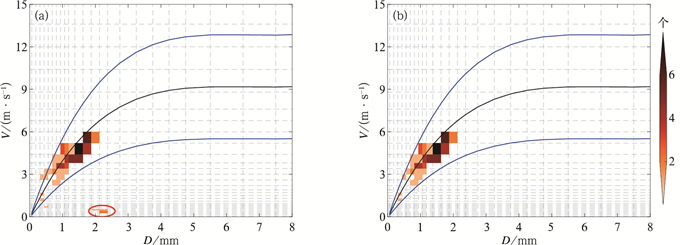图 1 分钟雨滴谱个数(填色)分布的速度质量控制(a)前、(b)后效果 [黑线为Brandes et al(2002)提出的经验速度，两条蓝线为±60%速度，红色圆圈内是待质量控制的粒子] Fig. 1 Velocity of (a) un-quality control and (b) quality control effects of one minute raindrop size distribution with drop counts with a number scale on the right [The black line indicates the Brandes et al (2002) empirical velocity and two blue lines represent the ±60% velocity, drops to be controlled are in the red circle]
1.2 雨滴谱分布计算公式

 $N\left({{D_i}} \right) = \sum\limits_{j = 1}^{32} {\frac{{{n_{ij}}}}{{A\Delta t{V_j}\Delta {D_i}}}}$ (3)

 ${Z = \sum\limits_{i = 1}^{32} N \left({{D_i}} \right){D^6}\Delta {D_i}}$ (4)
 ${R = \frac{{6{\rm{ \mathsf{ π} }}}}{{{{10}^4}}}\sum\limits_{i = 1}^{32} {\sum\limits_{j = 1}^{32} {\frac{{D_i^3{n_{ij}}}}{{A\Delta t}}} } }$ (5)
 ${W = \frac{{\rm{ \mathsf{ π} }}}{{6000}}{\rho _{\rm{w}}}\sum\limits_{i = 1}^{32} N \left({{D_i}} \right)D_i^3\Delta {D_i}}$ (6)

 $N(D) = {N_0}{D^\mu }\exp (- \mathit{\Lambda }D)$ (7)

 $M_{n}=\int_{0}^{\infty} D^{n} N(D) \mathrm{d} D$ (8)

 ${D_{\rm{m}}} = \frac{{{M_4}}}{{{M_3}}}$ (9)

 ${N_{\rm{w}}} = \frac{{{4^4}}}{{{\rm{ \mathsf{ π} }}{\rho _{\rm{w}}}}}\left({\frac{{{{10}^3}W}}{{D_{\rm{m}}^4}}} \right)$ (10)
1.3 雨滴谱和自动站数据对比

 $\delta = \frac{{\left| {PR{E_{\rm{Y}}} - PR{E_{{\rm{AWS}}}}} \right|}}{{PR{E_{{\rm{AWS}}}}}}$ (11)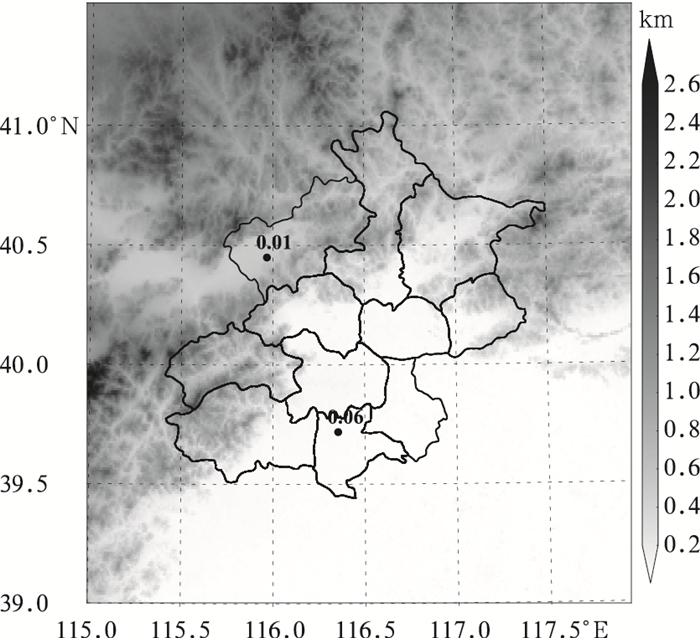图 2 北京地区海拔高度灰度图和选取的两站站点分布 (数字为相对误差) Fig. 2 Gray-scale map of altitude and the selected two sites in Beijing Region (number: relative error)
2 雨滴谱分布 2.1 分类方法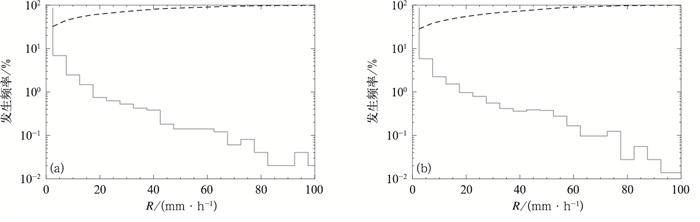图 3 (a) 延庆, (b)大兴站不同雨强降水的发生频率(实线)和对总雨量的累计贡献(虚线) Fig. 3 Frequency distribution of the rain with different intenstities (solid line) and accumulated contribution to the total rainfall (dashed line) of (a) Yanqing and (b) Daxing stations
2.2 分钟雨滴谱分布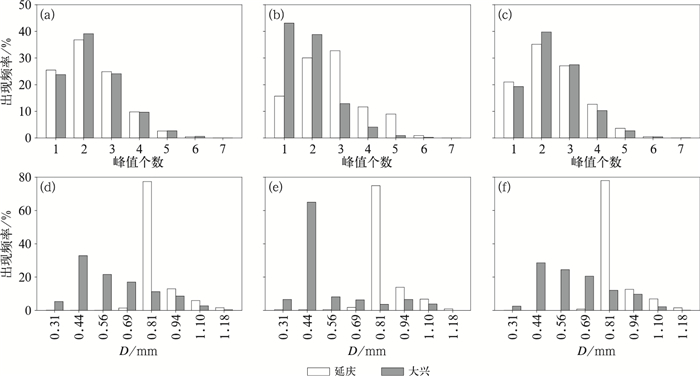图 4 延庆和大兴站(a，b，c)不同降水类型雨滴谱出现不同峰值的频率和(d，e，f)在各直径区间出现数浓度峰值的频率 (a, d)全部，(b, e)对流云，(c, f)层状云 Fig. 4 Frequency of different peaks in (a, b, c) raindrop spectra and (d, e, f) number concentration peaks in different diameter intervals of different rain types in Yanqing and Daxing stations (a, d) whole, (b, e) convective, (c, f) stratiform
2.3 平均谱分布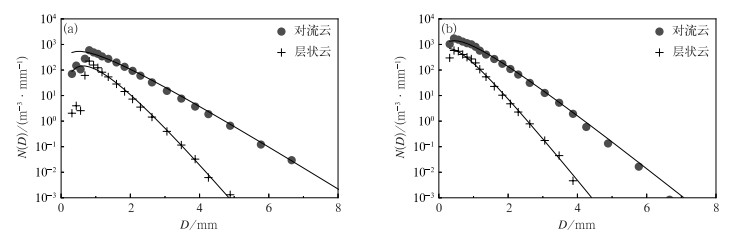图 5 (a) 延庆，(b)大兴站对流云和层状云降水平均谱及拟合Gamma分布曲线 Fig. 5 Composite raindrop spectra for convective and stratiform rain types, as well as the Gamma functions fitted on each spectrum using the truncated-moment method of (a) Yanqing and (b) Daxing stations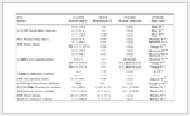表 1 平均谱的拟合Gamma分布参数 Table 1 The Gamma distribution parameters derived from the composite raindrop spectra using the truncated moment method
3 雨滴谱各参数分布特征 3.1 Dm-lgNw分布特征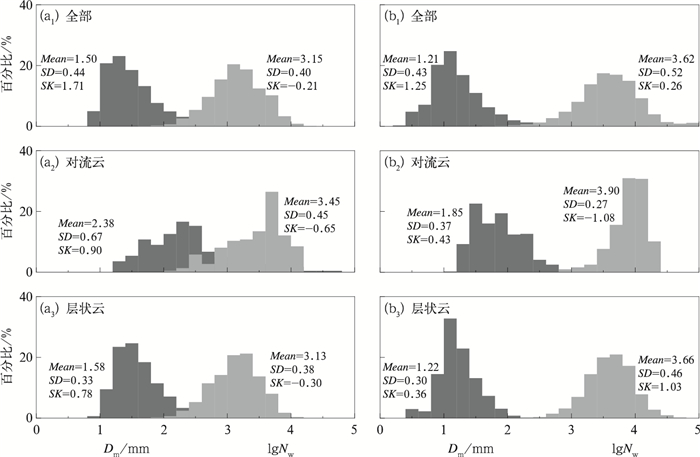图 6 (a) 延庆，(b)大兴站不同降水类型的Dm-lgNw直方图分布及相关统计参数(平均值、标准差和偏斜度) (a1，b1)全部，(a2，b2)对流云，(a3, b3)层状云 Fig. 6 Histogram of Dm-lgNw from different rain types and relative statistical parameters (Mean, SD, and SK) of (a) Yanqing and (b) Daxing stations (a1, b1) whole, (a2, b2) convective, (a3, b3) staritiform

(1) 两站的全部滴谱和层状云滴谱的Dm-lgNw分布特征较为一致，延庆站对流云(层状云)降水Dm和lgNw的平均值分别为2.38(1.58) mm和3.45(3.13) mm-1·m-3，大兴站对流云(层状云)降水Dm和lgNw的平均值分别为1.85(1.22) mm和3.90(3.66) mm-1·m-3。对流云滴谱相比于层状云滴谱，具有更大的质量平均直径(Dm)、标准化截断参数(lgNw)和分布谱宽。

(2) 延庆站，所有降水类型的Dm的斜度均为正值，lgNw的斜度均为负值，这与Chen et al(2013)得到的南京地区梅雨季节的滴谱特征相似，数值大小上，延庆站各谱均有更大的Dm和较小的lgNw，表明延庆站滴谱粒径更大，数浓度更小。大兴站，除了对流云lgNw偏斜度为负值，其他均为正值，表明各参数的频率分布主要集中在小值区。与Chen et al(2013)的结果相比，大兴站则体现出了粒径偏小，数浓度偏大的特征。

(3) 两站相比，延庆站的不同降水类型的Dm(lgNw)均大于(小于)大兴站，表明北京山区夏季降水有更多粒径较大的粒子和较低的数浓度，这可能与地形抬升导致的雨滴碰并、蒸发等过程相关。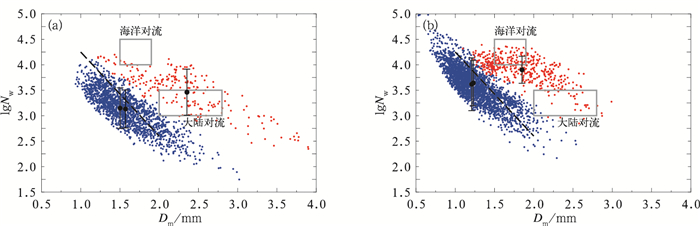图 7 (a) 延庆，(b)大兴站对流云(红点)、层状云(蓝点)降水Dm-lgNw散点分布 [黑色误差棒给出不同降水类型的平均Dm-lgNw和标准偏差，两灰色矩形框为Bringi et al(2003)提出的大陆和海洋对流范围，黑虚线为Bringi et al(2003)提出的层状云降水分界线] Fig. 7 Scatter plots of Dm-lgNw for convective (red) and stratiform (blue) rain types of (a) Yanqing and (b) Daxing stations [Black error bar gives the average Dm-lgNw and SD of different precipitation types, two gray rectangular boxes are the continental and martime convective ranges proposed by Bringi et al (2003), black dashed line is the stratiform boundary proposed by Bringi et al (2003)]
3.2 Dm-R关系和lgNw-R关系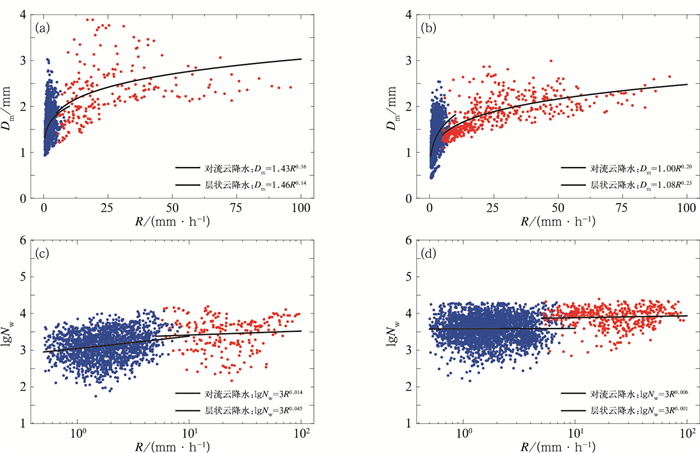图 8 (a, c)延庆，(b, d)大兴站对流云(红点)、层状云(蓝点)降水的(a，b)Dm-R、(c, d)lgNw-R散点图及拟合曲线(黑线) Fig. 8 Scatter plots of (a, b) Dm-R, (c, d) lgNw-R for convective (red) and stratiform (blue) rain types at (a, c) Yanqing and (b, d) Daxing stations (black line: fitted power-law relations)
3.3 μ-Λ关系

N0-μμ-Λ关系常被用于反演DSD特征，Brandes et al(2003)Zhang et al(2003)指出，和N0-μ关系相比，μ-Λ关系随气候和降水类型而变化，能够更好地反映真实滴谱情况。他们发现当R>5 mm·h-1时，佛罗里达地区夏季雨滴谱参数μΛ存在较好的二项式函数关系：

 $\mathit{\Lambda } = 0.0365{\mu ^2} + 0.735\mu + 1.935$ (12)

 $\mathit{\Lambda }=0.0141 \mu^{2}+0.550 \mu+1.776$ (13)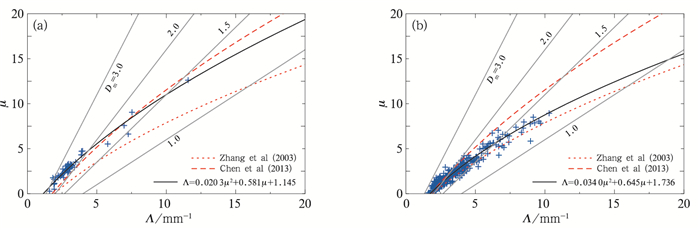图 9 (a) 延庆，(b)大兴站对流μ-Λ散点及拟合曲线(黑线) (灰线分别为Dm=1.0, 1.5, 2.0和3.0 mm对应的直线) Fig. 9 Scatter plots and fitted curves (black line) of μ-Λ values for convective rain of (a) Yanqing and (b) Daxing stations (Grey lines correspond to the values of Dm=1.0, 1.5, 2.0 and 3.0 mm given in ΛDm=4+μ)
 ${\mathit{\Lambda } = 0.0203{\mu ^2} + 0.581\mu + 1.145}$ (14)
 ${\mathit{\Lambda } = 0.0340{\mu ^2} + 0.645\mu + 1.736}$ (15)

3.4 Z-R关系分析

Z-R关系是雷达估测降水的基础，由滴谱分布差异引起的Z-R关系也随不同地区而变化，因此利用雨滴谱数据估测不同地区的Z-R关系效果更佳(汪学渊等，2016黄兴友等，2019)。Z=aRb关系的系数(a)和指数(b)与DSD的分布密切相关，按降水类型分布拟合关系，能够缩小ab值的变化范围(Rosenfeld and Ulbrich, 2003;刘红燕等，2008)，提高降水估测精度。图 10给出使用最小二乘法拟合两站滴谱Z-R关系的结果，作为参考，给出美国NEXRAD默认使用的代表夏季对流云降水的经典关系Z=300R1.40(Fulton et al，1998)和大陆层状云降水关系Z=200R1.60(Marshall and Palmer, 1948)。对延庆和大兴站的Z-R拟合关系分别为Z=764R1.20Z=386R1.32，各相关系数如表 2所示。从表 2可以看出，本文拟合的结果均好于或接近Z=300R1.40Z=200R1.60。其中Z=386R1.32的拟合曲线与Z=300R1.40基本重合，这与Ji et al(2019)分析北京滴谱得到的结论一致，表明北京平原地区的拟合关系式与经典关系Z=300R1.40较为接近；而代表山区的Z=764R1.20Z=300R1.40差别明显，拟合曲线位于经典关系上侧，表明给定Z，经典关系估测的R偏大，高估降水，不适合于北京山区。大陆层状云降水曲线在雨强较大时均位于两站滴谱拟合曲线上侧，表明给定Z，估测的R偏小，低估降水，同样不适合于北京地区夏季滴谱。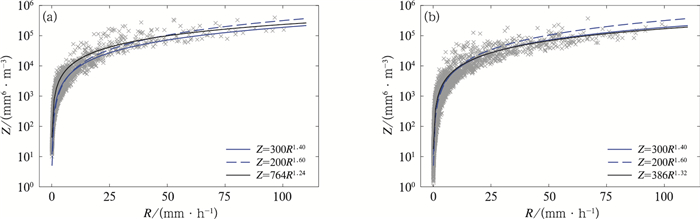图 10 (a) 延庆, (b)大兴站全部滴谱(灰色叉号)Z-R散点及拟合曲线(黑线) [蓝实线和虚线分别为Fulton et al(1998)的经典关系和Marshall and Palmer(1948)的大陆层状云降水关系] Fig. 10 Scatter plots (grey cross) and fitted curves (black line) of Z-R values for whole raindrop spectra of (a) Yanqing and (b) Daxing stations [Blue solid line and dashed line represent the standard Z-R relationship from Fulton et al (1998) and continental stratiform Z-R relationship from Marshall and Palmer (1948), respectively]表 2 延庆、大兴站各Z-R关系及相关系数 Table 2 The Z-R relationship and correlation coefficients at Yanqing and Daxing stations
4 结论与讨论

(1) 北京地区夏季降水的对流云滴谱含量较低，R≤5 mm·h-1的降水频次较多, 但对整体雨量贡献较小; 而R>5 mm·h-1的降水频次较少, 却对整体雨量贡献较大。分钟谱数据主要由双峰和多峰结构构成，粒子较为活跃；延庆、大兴站滴谱分别在0.812 mm、0.437 mm出现数浓度峰值。山区的滴谱数浓度峰值所在粒径更大。平均谱均为单峰结构，Gamma分布拟合效果较好，在小滴段存在一定误差。

(2) 对流云降水相比于层状云降水，具有更大的质量平均直径(Dm)、标准化截断参数(lgNw)和分布谱宽。两站相比，延庆站的不同降水类型的Dm(lgNw)均大于(小于)大兴站，表明北京地区山区滴谱粒径偏大数浓度偏小。与国外经典对流云滴谱相比，山区对流云降水滴谱倾向于大陆性对流，平原对流云降水滴谱倾向于海洋性对流。DmR增大而增大明显，并在大雨强时趋于稳定；lgNwR的指数关系不明显，但在Dm趋于稳定的大雨强时数值较大。

(3) 北京地区不同海拔高度的对流云降水滴谱μ-Λ关系均存在很好的二项拟合关系，山区对应更大的μDm。延庆站和大兴站的Z-R拟合关系分别为Z=764R1.20Z=386R1.32，其中大兴站的Z-R关系与代表夏季对流云降水的Z=300R1.40较为一致，而延庆站拟合的Z-R关系与Z=300R1.40存在一定差别，体现出北京平原和山区降水的差异。

(4) 与Chen et al(2013)研究的南京地区梅雨季节滴谱特征相比，北京夏季山区和平原的对流云降水和层状云降水含量均较低；且南京的Dm-lgNw特征介于北京两站之间，粒径分布上：延庆站最大，南京站次之，大兴站最小；数浓度分布上：大兴站最大，南京站次之，延庆站最小。